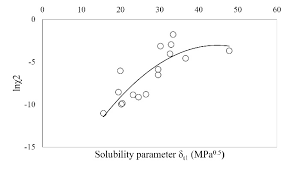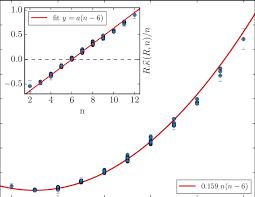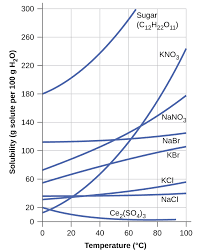## How to Calculate and Solve for Glass Gas Solubility Parameter in a Fluid | Nickzom CalculatorThe image above represents glass gas solubility parameter.

To compute for the glass gas solubility parameter, one essential parameter is needed and the parameter is Pressure (P).

The formula for calculating the glass gas solubility parameter:

x = 2.8869 – [14.1811 – 3.3093logP]0.5

Where:

x = Glass Gas Solubility Parameter, x
P = Pressure

Let’s solve an example;
Find the glass gas solubility parameter when the pressure is 62.

This implies that;

x = Glass Gas Solubility Parameter, x = 62

x = 2.8869 – [14.1811 – 3.3093logP]0.5
x = 2.8869 – [14.1811 – 3.3093log(62)]0.5
x = 2.8869 – [14.1811 – 3.3093(1.79)]0.5
x = 2.8869 – [14.1811 – 5.93]0.5
x = 2.8869 – [8.249]0.5
x = 2.8869 – 2.87
x = 0.0146

Therefore, the Glass Gas Solubility Parameter is 0.0146.

## How to Calculate and Solve for Mean Bubble Point in a Fluid | The Calculator EncyclopediaThe image above represents mean bubble point.

To compute for the mean bubble point, one essential parameter is needed and the parameter is Glass gas solubility parameter (x).

The formula for calculating mean bubble point:

Pb* = 10x

Where:

Pb* = Mean Bubble Point
x = Glass Gas Solubility Parameter

Let’s solve an example;
Given that the glass gas solubility parameter is 12. Find the mean bubble point?

This implies that;

x = Glass Gas Solubility Parameter = 12

Pb* = 10x
Pb* = 1012
Pb* = 1000000000000

Therefore, the Mean bubble point is 1000000000000.

## How to Calculate and Solve for Glass Gas Solubility in a Fluid | The Calculator EncyclopediaThe image above represents glass gas solubility.

To compute for the glass gas solubility, four essential parameters are needed and these parameters are API Gravity (°API), Temperature (°Rankine) (T), Mean Bubble Point (Pb*) and Gas Gravity at Actual Separator Psep and Tsepg).

The formula for calculating the glass gas solubility:

γgs = γg[((°API)0.989 / (T – 460)0.172)Pb*]1.2255

Where:

γgs = Glass Gas Solubility
°API = API Gravity
T = Temperature (°Rankine)
Pb* = Mean Bubble Point
γg = Gas Gravity at Actual Separator Psep and Tsep

Let’s solve an example;
Find the glass gas solubility when the API Gravity is 15, the temperature is 30, the mean bubble point is 40 and the gas gravity at actual separator is 54.

This implies that;

°API = API Gravity = 15
T = Temperature (°Rankine) = 30
Pb* = Mean Bubble Point = 40
γg = Gas Gravity at Actual Separator Psep and Tsep = 54

γgs = γg[((°API)0.989 / (T – 460)0.172)Pb*]1.2255
γgs = 54[((15)0.989 / (30 – 460)0.172)40]1.2255
γgs = 54[((15)0.989 / (-430)0.172)40]1.2255
γgs = 54[((15)0.989 / (NaN))40]1.2255
γgs = 54[(14.5 / NaN)40]1.2255
γgs = 54[(NaN)40]1.2255
γgs = 54[NaN]1.2255
γgs = 54[NaN]
γgs = NaN

Therefore, the glass gas solubility is NaN.